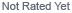Loading... Please wait...

# How much energy can a solar panel produce in a day?

## How much energy can a solar panel produce in a day?In our previous article “Understanding Energy and Power” we talked about the difference between power (measured in watts) and energy (measured in watt-hours). Energy is power happening over time. We showed you how to use Equation 1 to calculate the amount of energy (watt-hours) that a certain device will use in a certain period of time, once you know how much power (watts) that device uses while it is running.

Equation 1: ENERGY = POWER X TIME

Just as electrical devices are rated by the power that they use while they are running, solar panels are rated by the amount of power that they produce. If you look at the back of a solar panel, you will see a plate that lists the panel’s maximum power rating (usually abbreviated Wmp). You will see other things listed there too: One of them is the Vmp (the voltage of the panel at maximum power), and another is the Imp (the current of the panel at maximum power). In our 80 Watt Solar Panel the Vmp is 17.3V and the Imp is 4.7 amps. 17.3 X 4.7 = 81.3 Watts.
Here are some other panels if you want to compare: 10 Watt solar panel20 Watt Solar panel40 watt solar panel.

It would be nice and simple if you could just use Equation 1 to multiply the rated power Wmp (80 watts in this example) of a solar panel by the total number of hours of sunlight to calculate the energy that panel can produce in a day. But, you can’t, and here’s why:

1)First of all, the solar panel ratings are not really correct. The power output of a solar panel is highest when the panel is not powering anything, but that is not really such a useful number, is it? When the panel is hooked up to a load, the voltage drops as it powers the load, and the total power output of the panel is less than the rated power. Unfortunately, solar panels are rated by the power they produce while they are not powering anything. The quick solution to this problem is to use the rated amps (Imp) and ignore the Wmp or Max rated power (like the 80 Watts in our example). This is because your typical charging voltage is more like 13V. 13V X 4.7A = 61.1 watts, not 80 watts).

2)The second reason is that the power produced by a solar panel depends on how powerful the sunlight is, and the power of the sunlight changes throughout the day from morning to night, reaching a peak at noon. It also changes with the weather, as well as through the seasons, and is different at different locations on Earth at any given moment.

In order to produce consistent ratings for solar panels, the solar panels are tested under what is called a Peak Sun. A Peak Sun is defined as 1000 watts of sunlight per square meter of surface, and is approximately equal to the power of the sun at noon on a clear day at the equator.

For the rest of us, the rest of the time, the sun is less powerful than 1000 watts per square meter.

So what can you do to figure out how much energy a solar panel can produce in a day? Well, ignoring the fact that Wmp is not actually correct, we’re going to show you how to use that solar panel rating, and an average number of hours of Peak Sun, in Equation 1.

What makes this possible is the solar insolation maps published by the NREL (National Renewable Energy Lab). These maps show the average hours of Peak Sun for locations around the globe. These maps are available for the United States on our website - US Solar Radiation Maps.

Not wanting to seem TOO helpful, the units used in these maps are “kWh/m2.day”. Don’t worry about it, you can read the color-coded numbers as “Hours of Peak Sun per day”, and plug them into Equation 1 with your solar panel rating to calculate the amount of energy (in watt-hours) that a particular solar panel can produce in a day:

Imp * Peak Sun Hours/day * 13V = watt-hours/day

In our 80 Watt solar panel example:
4.7A * (5 hours as example) * 13V (estimate) = 305 watt-hours/day.

Now, just multiply this number by 30 (to get total watt-hours in a month), then divide by 1000 (to get kilowatt-hours) and you can compare this number to the kWh listed on your electric bill.

## New Products

•••••## Follow us on

Copyright 2021 Sundance Solar . All Rights Reserved.  Sitemap | Bigcommerce Premium Themes by PSDCenter